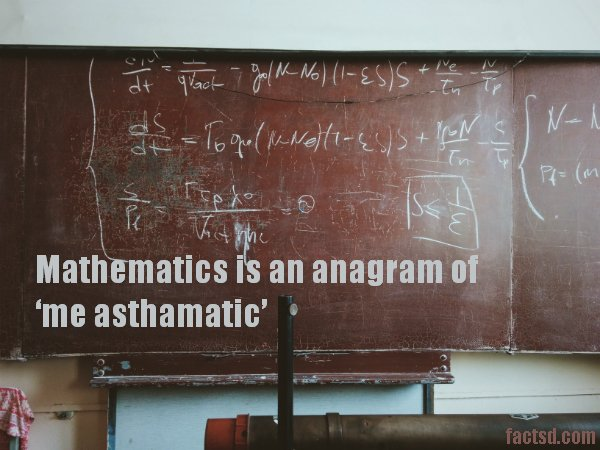# Math Facts – 65 Cool Facts About MathMath facts: Fun facts about math. The word mathematics itself scares the hell out of many. In school, math class is nothing less than a nightmare, but a close examination reveals that math is not that tough as it seems to be if one understands the logic and enjoys it. Math forms a significant part of our lives, it can be found everywhere in the universe, from daily chores to the stars high up in the sky. Hence learning math is prominent in life. Let’s explore some interesting facts about math.

## Math Facts

Most of the mathematical symbols were not invented until the 16th century, that’s why math equations were written in words, making it time consuming.

Ancient Babylonians used base 60 instead of 10 to solve math, therefore we have 60 seconds in 1 minute and 360 degrees in a circle.

The spiral shapes of sunflowers follow Fibonacci sequence, i.e. where you add preceding two numbers to get the next number in sequence, 1, 1, 2, 3, 5, 8, etc.

Students who chew gun have known to do better in math than those to do not.

The number that can’t be represented in Roman numerals is zero.

If a pack of cards are shuffled properly, there are chances that the exact order has never been seen in the entire history of the universe.

74% of girls in middle school express interest in technology, science, math and engineering, but when choosing a college major, just 0.4% of high school girls opt computer science.

The Millennium Prize is a \$1 million award given to the person who can solve any 1 of the 7 mathematical problems, but to date only 1 problem has been solved.

7 is the most popular number, the second popular is 3.

There is a condition called “Math Anxiety” that causes people to perform poorly in mathematics.